# Why I'm not wearing a mask (Fall 2021)

Since I started this blog in 2014, it has been entirely technical. Usually dedicated to examples that are intended to remind my future self and/or students of interesting problem solutions through code. However, as we return to in-person teaching in the Fall semester of 2021, under a strong recommendation to wear masks, I feel compelled to explain to my students why I won't be wearing one.

This won't be a commentary giving my opinion on the effectiveness of masks, nor a suggestion on whether any other individual should or should not wear one. This is simply documenting how I arrived at my decision to not wear a mask.

Before any vaccine was available to me, I caught and recovered from COVID-19. I confirmed that I have antibodies through a laboratory test in June of 2021. A summary of the results are shown below and the full report can be downloaded here.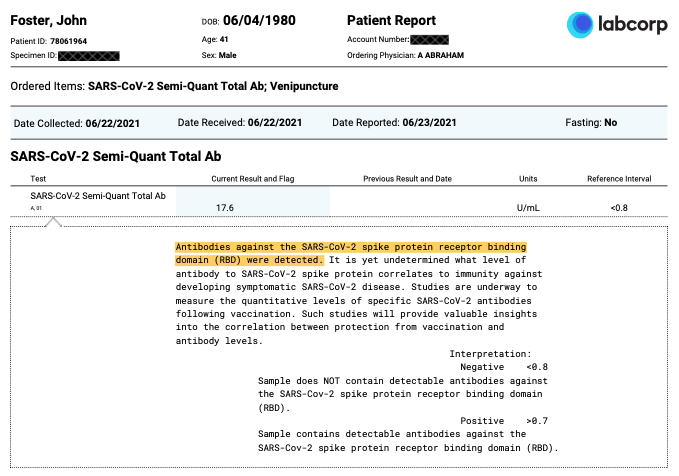This test is for antibodies against the receptor-binding-domain (RBD) of the SARS-CoV-2 spike protein (S-protein) which are known to wane over time; however, in response to recovery from infection the immune system will also produce other antibodies (e.g. N-protein), T-cells, B memory cells, etc. The number of RBD-specific memory B cells has been shown to remain unchanged after SARS-CoV-2 infection and have a resistance to RBD mutations (i.e. variants).

There are plenty of evidence-based findings that show that natural immunity is very robust. In this article by researchers at the Cleveland Clinic Health System, they follow over 50k employees and report

COVID-19 did not occur in anyone over the five months of the study among 2579 individuals previously infected with COVID-19, including 1359 who did not take the vaccine.

This report following >12,500 staff at the Oxford University Hospitals in the United Kingdom of which 1265 had positive antibody tests observed only 2 reinfections and they where both asymptomatic.

A recent Israel study reported that of 835,792 Israelis known to have recovered from COVID-19, there where only 72 known instances of reinfection (0.0086%) compared with over 3000 breakthrough infections of the 5,193,499 vaccinated (0.0578%) at the time of the report.

Finally, this last paper is not as statistically significant, but I think it’s interesting given the uniformity of the observation group. The paper Breakthrough Infections of SARS-CoV-2 Gamma Variant in Fully Vaccinated Gold Miners, French Guiana, 2021 with the main results summarized in this table describes a COVID outbreak among 44 gold miners, all men of roughly the same age and overall health status. Of the 44, 25 had been “fully vaccinated” and 9 had 1 dose — all Pfizer vaccines. Of the 25 fully vaccinated, 15 (!) had breakthrough infections. Of the 9 that had 1 dose, 6 had breakthrough infections. There were 6 that had recovered from COVID previously — 3 with 1 dose and 3 not vaccinated. Of those 6, none of them caught COVID in the outbreak.

So it appears that I should be immune, but for how long? There was this very recent paper in Nature, SARS-CoV-2 infection induces long-lived bone marrow plasma cells in humans that was then covered in this news article Had COVID? You’ll probably make antibodies for a lifetime that suggests a very long time, maybe forever.

Of course, these results should not be surprising, it's extremely rare to be infected twice by the same virus in a lifetime. The same paper linked above demonstrates

long-lasting T cells are reactive to the N protein of SARS-CoV 17 years after the outbreak of SARS in 2003; these T cells displayed robust cross-reactivity to the N protein of SARS-CoV-2.

Perhaps most interesting, this paper from 2008 demonstrates neutralizing antibody responses in 32 people (all of the participants) who had survived the 1918 H1N1 flu pandemic as children -- 90 years later!

Given these findings (and there are more, cf. Ref 6-8, and here, here, here, here, here, here, here, here, here, here, here, here, here, here), I concluded that my immunity to SARS-CoV-2 is strong and long-lived. However, there is a personal anecdote to share as well. In early August 2021, my wife visited a friend in Florida and 48 hours after her return she fell ill and tested positive for COVID-19. Two days after that her daughter, my step-daughter who lives with us, also became ill with COVID-19. Because I already had plenty of exposure to both of them in the house by the time we knew they were infected, I made no effort to quarantine myself from them and did what I could to make them comfortable. My wife's case was fairly mild with most of the symptoms subsiding after 5 days, my step-daughters case was very mild and she was completely recovered in 36 hours. After 8 days everyone was recovered with negative tests. I did not experience any symptoms or have any reason to believe I was infected during this time.

Given all the information above, I do not believe there is any medical reason for me to wear a mask while teaching at this time. For all practical purposes, you cannot catch a virus from which you are immune, and you certainly can't transmit a virus you don't have. I believe it's easier for the students to comprehend what I'm saying without a mask on, it's easier for me to project my voice without, and it's certainly more comfortable. I do plan to continue to monitor my antibody levels and may change my mind in the future.

UPDATE 11/10/2021: I had a second antibody test on 9/2/2021 and it showed that I my antibody levels remain strong, well above the threshold. The full report is available here.

Additionally, since writing this, there have been many more journal articles written on natural immunity that continue to demonstrate it is very robust. This website has been keeping a continuously updated list of references that, at the time of this update, is now up to 122 papers.

# What I might have done in a recent hackathon

### and why domain expertise matters in machine learning...¶

Recently, my colleague Prof. Michael Pyrcz (@GeostatsGuy) and myself hosted a data science hackathon open to students at The University of Texas at Austin with support from The Hildebrand Department of Petroleum and Geosystems Engineering. Given the COVID pandemic, we hosted the event virtually over two weekends in April 2021. The first weekend consisted of a workshop followed by an explanation of the problem dataset, then the students broke into teams and worked on their solutions that were ultimately submitted for scoring, code review, and presentation judging by a panel of industry experts the following weekend.

This was a really fun experience for me personally and the feedback has been incredible from all involved. Given that I have been teaching data science skills and challenging students in my classes with difficult coding exercises for years, it was great to see them showcase their capabilities.

I'd encourage you to take a look at the detailed problem statement and datasets linked to above, but briefly, the challenge was: given petrophysical data from well logs and spatial maps interpreted from seismic on 73 oil wells that have been in production for some time, predict the cumulative 3 year production for 10 new wells recently drilled, and having well log data, but not yet put into production. In addition to their "best estimate" the students where asked to provide 100 additional realizations such that an uncertainty model could be evaluated. The objective scoring consisted of comparisons of the mean squared error with respect to their "best estimate" and the true 3 year production (which we know, but was withheld from the students) as well as a scoring of their uncertainty model with a "goodness measure" proposed by Deutsch (1996).

I thought it would be a fun exercise to consider what I might have done to complete this challenge myself. In the spirit of the hackathon, I'll limit my time working on the project to what I can accomplish in a single day. Of course, since I am already familiar with the dataset, I have a head start over the teams in the competition who generally spent a few hours just investigating the data the first day. But given my short timeline, I won't be training any complicated neural network architectures or really any complicated machine learning models at all. I want to see how I can use my domain expertise to engineer meaningful features to get an answer quickly. I also have a surprise in store with respect to how I handled the uncertainty model. So let's get started...

### Feature Imputation¶

First, like all work in Python, I'll start with the module imports used during this work.

In :
import pandas as pd
import seaborn as sns
import numpy as np
import matplotlib.pyplot as plt
from matplotlib import cm
from matplotlib import colors
from scipy.optimize import curve_fit
from sklearn.cluster import KMeans
from sklearn.neighbors import KNeighborsClassifier
from sklearn.model_selection import GridSearchCV, KFold
from sklearn.decomposition import PCA
from sklearn.preprocessing import (PolynomialFeatures, MaxAbsScaler,
MinMaxScaler, StandardScaler, RobustScaler)
from sklearn.linear_model import LinearRegression, ElasticNet
from sklearn.tree import DecisionTreeRegressor
from sklearn.ensemble import BaggingRegressor
from sklearn.pipeline import Pipeline
from sklearn.metrics import mean_absolute_percentage_error
sns.set(rc={'text.usetex' : True})


And I'll read in the well log datasets from both the wells that have been in production as well as what we call the preproduction wells, i.e. those we are trying to predict the cumulative production for after 3 years.

In :
production_wells_df = pd.read_csv('wellbore_data_producer_wells.csv',
index_col='Well_ID')
index_col='Well_ID')


Now we'll combine those two dataframes into a single dataframe.

In :
wells_df = pd.concat([production_wells_df, preproduction_wells_df])

Out:
X, m Y, m Depth, m Porosity, fraction Permeability, mD Acoustic Impedance, kg/s-m^2 Rock facies Density, g/cm3 Compressible velocity, m/s Youngs modulus, GPa Shear velocity, m/s Shear modulus, GPa
Well_ID
Well_no_1 7325 7175 3052.8 0.13330 NaN 6981171.853 Sandstone 2.280137 3106.544655 24.721555 1690.417133 7.304717
Well_no_1 7325 7175 3053.3 0.13865 NaN 7234748.871 Sandstone 2.135061 4003.697087 23.360728 1573.847967 5.202120
Well_no_1 7325 7175 3053.8 0.14638 NaN 7157383.755 Sandstone 1.991045 3462.569030 28.232152 1636.279139 NaN
Well_no_1 7325 7175 3054.3 NaN NaN NaN Sandstone 1.694242 3836.960702 29.220132 1613.043048 5.074763
Well_no_1 7325 7175 3054.8 0.14993 NaN NaN Sandstone 1.664371 3919.585777 NaN 1636.846284 5.277834

And inspect some overall statistics on the dataset.

In :
wells_df.describe().T

Out:
count mean std min 25% 50% 75% max
X, m 1660.0 4.573795e+03 1982.122873 1.175000e+03 2.975000e+03 4.275000e+03 6.225000e+03 9.025000e+03
Y, m 1660.0 5.618373e+03 2284.982172 7.750000e+02 3.975000e+03 5.675000e+03 7.525000e+03 9.775000e+03
Depth, m 1660.0 3.055563e+03 4.282596 3.045620e+03 3.052505e+03 3.055550e+03 3.058573e+03 3.066300e+03
Porosity, fraction 1444.0 1.278649e-01 0.036297 4.027000e-02 1.138025e-01 1.376300e-01 1.526325e-01 1.889100e-01
Permeability, mD 332.0 1.140680e+02 81.300030 2.214604e+00 5.165015e+01 9.479379e+01 1.558035e+02 4.892194e+02
Acoustic Impedance, kg/s-m^2 1544.0 7.323882e+06 312125.112401 6.559277e+06 7.105710e+06 7.271181e+06 7.502261e+06 8.249272e+06
Density, g/cm3 1411.0 2.050372e+00 0.414102 1.421943e+00 1.734559e+00 1.988778e+00 2.230457e+00 3.530373e+00
Compressible velocity, m/s 1444.0 3.688528e+03 724.061269 1.661828e+03 3.172568e+03 3.663243e+03 4.215650e+03 6.179653e+03
Youngs modulus, GPa 1428.0 2.731047e+01 5.454678 1.320513e+01 2.343858e+01 2.661773e+01 3.092854e+01 4.812329e+01
Shear velocity, m/s 1444.0 1.675224e+03 99.491202 1.307887e+03 1.608163e+03 1.674237e+03 1.744162e+03 1.989829e+03
Shear modulus, GPa 1461.0 5.759466e+00 1.501610 1.650985e+00 4.762268e+00 5.642379e+00 6.609150e+00 1.182004e+01

We can see that there is quite a bit of missing data. Of particular concern is the large amount of missing permeabilities as this will be one of the strongest indicators of production. So our first task is going to be to impute the missing features, especially working toward a good model for permeability. To start, let's plot some of the provided map data. Here we are showing the spatial distributions of percentage facies in the reservoir. There is a fault in the reservoir which we only know the location of, but no other information. The white circles represent wells we have production for, the red circles are wells we are trying to predict production. The fault is indicated by the red line.

In :
production_well_locations = \
(production_wells_df[['X, m', 'Y, m']]
.groupby(production_wells_df.index)
.mean())
preproduction_well_locations = \
(preproduction_wells_df[['X, m', 'Y, m']]
.groupby(preproduction_wells_df.index)
.mean())
px = production_well_locations['X, m'].to_numpy()[::1]
py = -production_well_locations['Y, m'].to_numpy() + 10000
ppx = preproduction_well_locations['X, m'].to_numpy()[::1]
ppy = -preproduction_well_locations['Y, m'].to_numpy() + 10000
fx = np.linspace(0, 1e4, num=5)
fy = fx - 1750
fig = plt.figure(constrained_layout=True, figsize=(8,5))
ax = fig.subplot_mosaic(
[['sand',  'sandy shale', 'colorbar'],
['shale', 'shaly sand',  'colorbar']],
gridspec_kw={"width_ratios": [1, 1, 0.1]})
cmap = cm.get_cmap('viridis', 100)
bounds = np.linspace(0,1.0,num=100)
vals = bounds#[:-1]
norm = colors.BoundaryNorm(bounds, cmap.N)
extent=[0, 10000, 0, 10000])
ax['sand'].scatter(px, py, color='w', marker='o', alpha=0.5)
ax['sand'].scatter(ppx, ppy, color='r', marker='o', alpha=0.5)
ax['sand'].plot(fx, fy, color='r')
ax['sand'].set_ylim([0,1e4])
ax['sand'].set_title('Sand')
ax['sand'].set_aspect(1)
ax['sand'].grid(None)
norm=norm, extent=[0, 10000, 0, 10000])
ax['sandy shale'].scatter(px, py, color='w', marker='o', alpha=0.5)
ax['sandy shale'].scatter(ppx, ppy, color='r', marker='o', alpha=0.5)
ax['sandy shale'].plot(fx, fy, color='r')
ax['sandy shale'].set_ylim([0,1e4])
ax['sandy shale'].set_title('Sandy shale')
ax['sandy shale'].set_aspect(1)
ax['sandy shale'].grid(None)
extent=[0, 10000, 0, 10000])
ax['shale'].scatter(px, py, color='w', marker='o', alpha=0.5)
ax['shale'].scatter(ppx, ppy, color='r', marker='o', alpha=0.5)
ax['shale'].plot(fx, fy, color='r')
ax['shale'].set_ylim([0,1e4])
ax['shale'].set_title('Shale')
ax['shale'].set_aspect(1)
ax['shale'].grid(None)
norm=norm, extent=[0, 10000, 0, 10000])
ax['shaly sand'].scatter(px, py, color='w', marker='o', alpha=0.5)
ax['shaly sand'].scatter(ppx, ppy, color='r', marker='o', alpha=0.5)
ax['shaly sand'].plot(fx, fy, color='r')
ax['shaly sand'].set_ylim([0,1e4])
ax['shaly sand'].set_title('Shaly sand')
ax['shaly sand'].set_aspect(1)
ax['shaly sand'].grid(None)
cbar = fig.colorbar(im, cax=ax['colorbar'], boundaries=bounds, values=vals);
cbar.set_ticks([0.0, 0.25, 0.5, 0.75, 1.0])
cbar.set_ticklabels([r'$0$', r'$\frac{1}{4}$', r'$\frac{1}{2}$',
r'$\frac{3}{4}$', r'$1$'])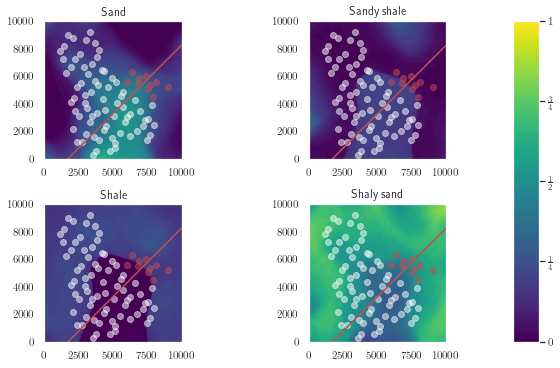It doesn't look like the fault produces any offset or discontinuity in the spatial facies information, that's good news, so we'll proceed with imputing the missing facies information using spatial location information. First, we'll subset the dataframe and replace the string labels for facies with numerical ones, so 0 will indicated Sandstone, 1 will indicate Sandy shale, and so on...

In :
facies_df = (wells_df[['X, m', 'Y, m', 'Depth, m', 'Rock facies']]
.replace({'Sandstone': 0,
'Sandy shale': 1,
'Shale': 2,
'Shaly sandstone': 3})
)
missing_facies = facies_df['Rock facies'].isnull()

Out:
X, m Y, m Depth, m Rock facies
Well_ID
Well_no_1 7325 7175 3052.8 0.0
Well_no_1 7325 7175 3053.3 0.0
Well_no_1 7325 7175 3053.8 0.0
Well_no_1 7325 7175 3054.3 0.0
Well_no_1 7325 7175 3054.8 0.0

Now we'll build a k-nearest neighbors classifier, do a little hyperparameter tuning with scikit-learn's builtin GridSearchCV.

In :
parameters = {'weights': ('uniform', 'distance'),
'n_neighbors':[4, 6, 8, 10]}
knn = KNeighborsClassifier()
gcv = GridSearchCV(knn, parameters, cv=KFold(random_state=2, shuffle=True))
gcv.fit(facies_df.loc[~missing_facies, 'X, m':'Depth, m'],
facies_df.loc[~missing_facies, 'Rock facies'])
gcv.best_params_

Out:
{'n_neighbors': 4, 'weights': 'distance'}

Using the hyperparameter settings above, we can now predict (and impute) the missing facies values.

In :
facies_df.loc[missing_facies, 'Rock facies'] = \
gcv.predict(facies_df.loc[missing_facies, 'X, m':'Depth, m'])
wells_df['Rock facies'] = facies_df['Rock facies'].astype('int')
wells_df.describe().T.loc[['X, m', 'Y, m', 'Rock facies']]

Out:
count mean std min 25% 50% 75% max
X, m 1660.0 4573.795181 1982.122873 1175.0 2975.0 4275.0 6225.0 9025.0
Y, m 1660.0 5618.373494 2284.982172 775.0 3975.0 5675.0 7525.0 9775.0
Rock facies 1660.0 1.227711 1.335725 0.0 0.0 1.0 3.0 3.0

Given that we'd expect rocks of the same facies to have a similar density and acoustic impedance, we'll impute those missing features with the averages from each facies.

In :
wells_df['Density, g/cm3'] = \
(wells_df.groupby('Rock facies')['Density, g/cm3']
.apply(lambda df: df.fillna(df.mean()))
.reset_index()
.set_index(['Well_ID'])
)

wells_df['Acoustic Impedance, kg/s-m^2'] = \
(wells_df.groupby('Rock facies')['Acoustic Impedance, kg/s-m^2']
.apply(lambda df: df.fillna(df.mean()))
.reset_index()
.set_index(['Well_ID'])
)


Now we'll subset the dataframe and use the features shown to impute the missing porosity values using polynomial regression.

In :
missing_porosity = wells_df['Porosity, fraction'].isnull()
porosity_df = (wells_df.loc[:, 'X, m':'Density, g/cm3']
.drop('Permeability, mD', axis=1))

Out:
X, m Y, m Depth, m Porosity, fraction Acoustic Impedance, kg/s-m^2 Rock facies Density, g/cm3
Well_ID
Well_no_1 7325 7175 3052.8 0.13330 6.981172e+06 0 2.280137
Well_no_1 7325 7175 3053.3 0.13865 7.234749e+06 0 2.135061
Well_no_1 7325 7175 3053.8 0.14638 7.157384e+06 0 1.991045
Well_no_1 7325 7175 3054.3 NaN 7.124201e+06 0 1.694242
Well_no_1 7325 7175 3054.8 0.14993 7.124201e+06 0 1.664371

We'll setup a pipeline and use GridSearchCV again to find the best hyperparameters.

In :
scalers = [MaxAbsScaler(), MinMaxScaler(), StandardScaler(), RobustScaler()]

pipe = Pipeline([
('scaler', StandardScaler()),
('pca', PCA()),
('poly', PolynomialFeatures()),
('reg', LinearRegression())
])

params = {
'scaler': scalers,
'poly__degree': [1, 2, 3],
'pca__n_components': [1, 2, 3, 4, 5, 6],
}

gcv = GridSearchCV(pipe, params, cv=KFold(random_state=5, shuffle=True))
gcv.fit(porosity_df[~missing_porosity].drop('Porosity, fraction', axis=1),
porosity_df.loc[~missing_porosity, 'Porosity, fraction'])
gcv.best_params_

Out:
{'pca__n_components': 5, 'poly__degree': 2, 'scaler': MinMaxScaler()}

The best parameters are shown above. Now we'll use this model to impute the missing porosity.

In :
porosity_df.loc[missing_porosity, 'Porosity, fraction'] = \
gcv.predict(porosity_df[missing_porosity].drop('Porosity, fraction', axis=1))


Below we'll plot the imputed and given porosities. Nothing looks too strange here, none of the imputed values are outliers with respect to the ranges of the given data.

In :
fig, ax = plt.subplots()
ax.scatter(porosity_df[~missing_porosity &
(porosity_df['Rock facies'] == 0)]['Depth, m'],
porosity_df[~missing_porosity &
(porosity_df['Rock facies'] == 0)]['Porosity, fraction'],
color='r', facecolors='none', label='Sandstone')
ax.scatter(porosity_df[missing_porosity &
(porosity_df['Rock facies'] == 0)]['Depth, m'],
porosity_df[missing_porosity &
(porosity_df['Rock facies'] == 0)]['Porosity, fraction'],
color='r', label='Sandstone (Imputed)')
ax.scatter(porosity_df[~missing_porosity &
(porosity_df['Rock facies'] == 1)]['Depth, m'],
porosity_df[~missing_porosity &
(porosity_df['Rock facies'] == 1)]['Porosity, fraction'],
color='b', facecolors='none', label='Sandy shale')
ax.scatter(porosity_df[missing_porosity &
(porosity_df['Rock facies'] == 1)]['Depth, m'],
porosity_df[missing_porosity &
(porosity_df['Rock facies'] == 1)]['Porosity, fraction'],
color='b', label='Sandy shale (Imputed)')
ax.scatter(porosity_df[~missing_porosity &
(porosity_df['Rock facies'] == 2)]['Depth, m'],
porosity_df[~missing_porosity &
(porosity_df['Rock facies'] == 2)]['Porosity, fraction'],
color='g', facecolors='none', label='Shale')
ax.scatter(porosity_df[missing_porosity &
(porosity_df['Rock facies'] == 2)]['Depth, m'],
porosity_df[missing_porosity &
(porosity_df['Rock facies'] == 2)]['Porosity, fraction'],
color='g', label='Shale (Imputed)')
ax.scatter(porosity_df[~missing_porosity &
(porosity_df['Rock facies'] == 3)]['Depth, m'],
porosity_df[~missing_porosity &
(porosity_df['Rock facies'] == 3)]['Porosity, fraction'],
color='k', facecolors='none', label='Shaly sand')
ax.scatter(porosity_df[missing_porosity &
(porosity_df['Rock facies'] == 3)]['Depth, m'],
porosity_df[missing_porosity &
(porosity_df['Rock facies'] == 3)]['Porosity, fraction'],
color='k', label='Shaly sand (Imputed)')
ax.set_xlabel('Depth, m')
ax.set_ylabel('Porosity, fraction')
ax.legend(bbox_to_anchor=(1.5, 1), loc='upper right', ncol=1);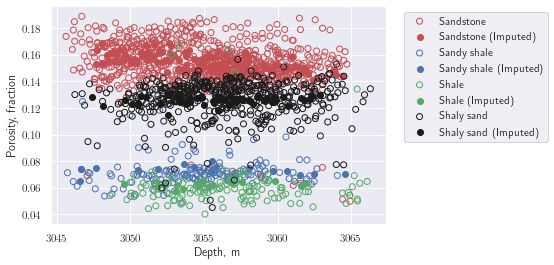We can also look at the distribution of the imputed porosities and compare to the given values. The imputation preserves the bimodal distribution of the original data.

In :
fig, ax = plt.subplots(1, 2)
sns.distplot(porosity_df.loc[~missing_porosity, 'Porosity, fraction'], ax=ax)
ax.set_title('Given values')
sns.distplot(porosity_df.loc[missing_porosity, 'Porosity, fraction'], ax=ax);
sns.distplot(porosity_df.loc[missing_porosity, 'Porosity, fraction'], ax=ax);
ax.set_title('Imputed values');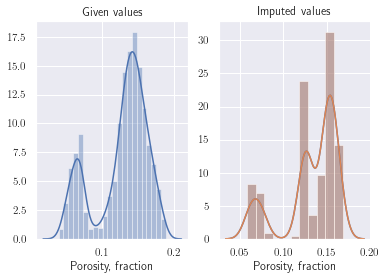Now we'll add this imputed data to our wells_df.

In :
wells_df['Porosity, fraction'] =  porosity_df['Porosity, fraction']


To impute the missing permeabilities, we'll use some knowledge of petrophysics to do a little feature engineering. There is a widely used correlation between porosity and permeability called the Kozeny-Carmen relationship which models permeability as

$$\kappa \sim \frac{\phi^3}{(1-\phi)^2}$$

where $\kappa$ is the permeability and $\phi$ is the porosity. We can quickly take a look at this relationship using seaborn's builtin regplot function, i.e.

In :
missing_perms = wells_df['Permeability, mD'].isnull()
ϕ = wells_df.loc[~missing_perms, 'Porosity, fraction'].to_numpy()
fig, ax = plt.subplots()
sns.regplot(ϕ ** 3 / (1 - ϕ) ** 2,
wells_df.loc[~missing_perms, 'Permeability, mD'], ax=ax);
ax.set_xlabel(r'$\frac{\phi^3}{(1 - \phi)^2}$');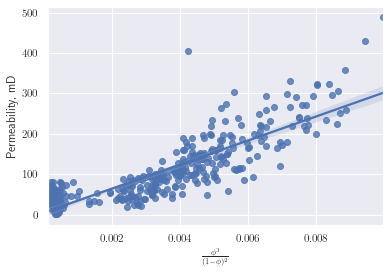While not the best model, we can use this data to condition our more complex prediction to come. First we find the slope and intercept of the blue line above.

In :
fphi = lambda ϕ, m, kappa0: m * ϕ ** 3 / (1 - ϕ) ** 2 + kappa0
popt, _ = curve_fit(fphi, ϕ, wells_df.loc[~missing_perms, 'Permeability, mD'])


Now we'll use the model to create a feature we'll call 'KC permeability, mD'.

In :
perm_df = wells_df.loc[:, 'X, m':'Density, g/cm3']
perm_df['KC permeability, mD'] = fphi(wells_df['Porosity, fraction'],
popt, popt)


Using the data show above, we'll build a model to impute the missing permeabilities. Again, using GridSearchCV to hyperparameter tune, we have

In :
scalers = [MaxAbsScaler(), MinMaxScaler(), StandardScaler(), RobustScaler()]

pipe = Pipeline([
('scaler', StandardScaler()),
('pca', PCA()),
('poly', PolynomialFeatures()),
('reg', LinearRegression())
])

params = {
'scaler': scalers,
'poly__degree': [2, 3, 4],
'pca__n_components': [1, 2, 3, 4, 5, 6, 7],
}

gcv = GridSearchCV(pipe, params, cv=KFold(random_state=5, shuffle=True))
gcv.fit(perm_df[~missing_perms].drop('Permeability, mD', axis=1),
perm_df.loc[~missing_perms, 'Permeability, mD'])
gcv.best_params_

Out:
{'pca__n_components': 4, 'poly__degree': 2, 'scaler': RobustScaler()}

With these parameters, we can predict the missing permeabilities.

In :
perm_df.loc[missing_perms, 'Permeability, mD'] = \
gcv.predict(perm_df[missing_perms].drop('Permeability, mD', axis=1))


Visualizing the results of the prediction against the given data, this model appears to perform well.

In :
fig, ax = plt.subplots()
ax.scatter(perm_df[~missing_perms &
(perm_df['Rock facies'] == 0)]['Porosity, fraction'],
perm_df[~missing_perms &
(perm_df['Rock facies'] == 0)]['Permeability, mD'],
color='r', facecolors='none', label='Sandstone')
ax.scatter(perm_df[missing_perms &
(perm_df['Rock facies'] == 0)]['Porosity, fraction'],
perm_df[missing_perms &
(perm_df['Rock facies'] == 0)]['Permeability, mD'],
color='r', label='Sandstone (Imputed)')
ax.scatter(perm_df[~missing_perms &
(perm_df['Rock facies'] == 1)]['Porosity, fraction'],
perm_df[~missing_perms &
(perm_df['Rock facies'] == 1)]['Permeability, mD'],
color='b', facecolors='none', label='Sandy shale')
ax.scatter(perm_df[missing_perms &
(perm_df['Rock facies'] == 1)]['Porosity, fraction'],
perm_df[missing_perms &
(perm_df['Rock facies'] == 1)]['Permeability, mD'],
color='b', label='Sandy shale (Imputed)')
ax.scatter(perm_df[~missing_perms &
(perm_df['Rock facies'] == 2)]['Porosity, fraction'],
perm_df[~missing_perms &
(perm_df['Rock facies'] == 2)]['Permeability, mD'],
color='g', facecolors='none', label='Shale')
ax.scatter(perm_df[missing_perms &
(perm_df['Rock facies'] == 2)]['Porosity, fraction'],
perm_df[missing_perms &
(perm_df['Rock facies'] == 2)]['Permeability, mD'],
color='g', label='Shale (Imputed)')
ax.scatter(perm_df[~missing_perms &
(perm_df['Rock facies'] == 3)]['Porosity, fraction'],
perm_df[~missing_perms &
(perm_df['Rock facies'] == 3)]['Permeability, mD'],
color='k', facecolors='none', label='Shaly sand')
ax.scatter(perm_df[missing_perms &
(perm_df['Rock facies'] == 3)]['Porosity, fraction'],
perm_df[missing_perms &
(perm_df['Rock facies'] == 3)]['Permeability, mD'],
color='k', label='Shaly sand (Imputed)')
ax.set_xlabel('Porosity, fraction')
ax.set_ylabel('Permeability, mD')
ax.legend(bbox_to_anchor=(1.5, 1), loc='upper right', ncol=1);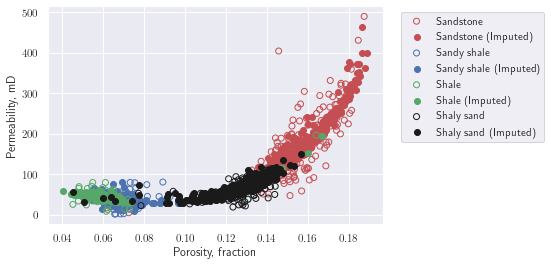The 'KC permeability, mD' is redundant now, so we'll drop it from the dataframe.

In :
perm_df.drop('KC permeability, mD', axis=1, inplace=True)


### Feature engineering¶

Since the fault runs right through both the produced wells and the wells we are trying to predict production for, let's engineer a few features related to the fault. First we'll engineer a feature we'll call 'Distance to fault' which computes the perpendicular distance to the fault. Also, we don't know if the fault compartmentalizes the reservoir in any way, so we'll also create a feature called 'left/right fault' to indicate which side of the fault a well lies.

In :
perm_df['Distance to fault'] = np.sqrt((perm_df['X, m'] + perm_df['Y, m'] - 11750) ** 2) / np.sqrt(2)
perm_df['left/right fault'] = np.sign(perm_df['X, m'] * (-11750) - (perm_df['Y, m'] - 11750) * (11750))


Because all of the prediction wells are "near" the fault, we'll create another boolean feature that gives some importance to wells near the fault. We'll define "near" as any well that is closer than the mean preproduction (i.e. prediction) well distance from the fault.

In :
preproduction_wells = perm_df.index.isin(preproduction_wells_df
.index.to_numpy())
max_distance = perm_df.loc[preproduction_wells, 'Distance to fault'].mean()
perm_df['close to fault'] = np.where(
perm_df['Distance to fault'] < max_distance, 1, 0)


Give that we anticipate wells that are "similar" to have "similar" production, we'll use KMeans clustering to find similar wells in our dataframe

In :
scalers = [MaxAbsScaler(), MinMaxScaler(), StandardScaler(), RobustScaler()]

pipe = Pipeline([
('scaler', StandardScaler()),
('cluster', KMeans())
])

params = {
'scaler': scalers,
'cluster__n_clusters': [10, 12, 14],
}

gcv = GridSearchCV(pipe, params, cv=KFold(random_state=3, shuffle=True))
gcv.fit(perm_df.loc[~preproduction_wells])
gcv.best_params_

Out:
{'cluster__n_clusters': 14, 'scaler': MaxAbsScaler()}

And use the model to predict which cluster the preproduction wells would fall into.

In :
perm_df['Cluster'] = gcv.predict(perm_df)


Now we'll read in the production histories and merge them with our working dataframe.

In :
production_history_df = pd.read_csv('production_history.csv',
index_col='Well_ID')
production_df = perm_df.merge(production_history_df,
how='left',
left_index=True,
right_index=True)

Out:
X, m Y, m Depth, m Porosity, fraction Permeability, mD Acoustic Impedance, kg/s-m^2 Rock facies Density, g/cm3 Distance to fault left/right fault close to fault Cluster Cumulative oil production (1 yr), MSTB Cumulative oil production (2 yr), MSTB Cumulative oil production (3 yr), MSTB Cumulative Water production (1 yr), MSTB Cumulative Water production (2 yr), MSTB Cumulative Water production (3 yr), MSTB
Well_ID
Well_no_1 7325 7175 3052.8 0.133300 84.088999 6.981172e+06 0 2.280137 1944.543648 -1 0 4 450.98 684.2 847.33 494.89 1236.4 2108.7
Well_no_1 7325 7175 3053.3 0.138650 94.107409 7.234749e+06 0 2.135061 1944.543648 -1 0 4 450.98 684.2 847.33 494.89 1236.4 2108.7
Well_no_1 7325 7175 3053.8 0.146380 117.272922 7.157384e+06 0 1.991045 1944.543648 -1 0 4 450.98 684.2 847.33 494.89 1236.4 2108.7
Well_no_1 7325 7175 3054.3 0.156552 151.990789 7.124201e+06 0 1.694242 1944.543648 -1 0 4 450.98 684.2 847.33 494.89 1236.4 2108.7
Well_no_1 7325 7175 3054.8 0.149930 124.505979 7.124201e+06 0 1.664371 1944.543648 -1 0 4 450.98 684.2 847.33 494.89 1236.4 2108.7

Now we'll compute the average 1, 2, and 3 year productions for each cluster and assign a new feature with this average value as the expected average production at each well location.

In :
avg_production_df = (production_df
.groupby('Cluster')
.mean()
.loc[:, 'Cumulative oil production (1 yr), MSTB' \
:'Cumulative Water production (3 yr), MSTB']
.reset_index()
.rename(columns={'Cumulative oil production (1 yr), ' \
'MSTB':'Avg. oil prod (1 yr)',
'Cumulative oil production (2 yr), ' \
'MSTB':'Avg. oil prod (2 yr)',
'Cumulative oil production (3 yr), ' \
'MSTB':'Avg. oil prod (3 yr)',
'Cumulative Water production (1 yr), ' \
'MSTB':'Avg. water prod (1 yr)',
'Cumulative Water production (2 yr), ' \
'MSTB':'Avg. water prod (2 yr)',
'Cumulative Water production (3 yr), ' \
'MSTB':'Avg. water prod (3 yr)'})
)


Merging these averaged production values into our working dataframe, we have the final dataframe which we'll use to make predictions with.

In :
final_df = (production_df
.reset_index()
.merge(avg_production_df,
how='left',
on='Cluster')
.set_index(['Well_ID'])
)

Out:
X, m Y, m Depth, m Porosity, fraction Permeability, mD Acoustic Impedance, kg/s-m^2 Rock facies Density, g/cm3 Distance to fault left/right fault ... Cumulative oil production (3 yr), MSTB Cumulative Water production (1 yr), MSTB Cumulative Water production (2 yr), MSTB Cumulative Water production (3 yr), MSTB Avg. oil prod (1 yr) Avg. oil prod (2 yr) Avg. oil prod (3 yr) Avg. water prod (1 yr) Avg. water prod (2 yr) Avg. water prod (3 yr)
Well_ID
Well_no_1 7325 7175 3052.8 0.133300 84.088999 6.981172e+06 0 2.280137 1944.543648 -1 ... 847.33 494.89 1236.4 2108.7 510.514519 795.43159 1000.623598 562.638577 1375.202887 2333.438494
Well_no_1 7325 7175 3053.3 0.138650 94.107409 7.234749e+06 0 2.135061 1944.543648 -1 ... 847.33 494.89 1236.4 2108.7 510.514519 795.43159 1000.623598 562.638577 1375.202887 2333.438494
Well_no_1 7325 7175 3053.8 0.146380 117.272922 7.157384e+06 0 1.991045 1944.543648 -1 ... 847.33 494.89 1236.4 2108.7 510.514519 795.43159 1000.623598 562.638577 1375.202887 2333.438494
Well_no_1 7325 7175 3054.3 0.156552 151.990789 7.124201e+06 0 1.694242 1944.543648 -1 ... 847.33 494.89 1236.4 2108.7 510.514519 795.43159 1000.623598 562.638577 1375.202887 2333.438494
Well_no_1 7325 7175 3054.8 0.149930 124.505979 7.124201e+06 0 1.664371 1944.543648 -1 ... 847.33 494.89 1236.4 2108.7 510.514519 795.43159 1000.623598 562.638577 1375.202887 2333.438494

5 rows × 24 columns

### Predictions¶

In order to hyperparameter tune our forthcoming models, we're going to use the "goodness" measure of Deutsch, 1996, i.e.

$$\mbox{goodness} = 1 - \int_0^1 \left(2 a(p) - 2 \right) \left(\overline{\xi(p)} - p \right) \mathrm{d}p$$

in addition to the mean_absolute_percentage_error from scikit-learn to score our models. The idea will be to use the BaggingRegressor to create an ensemble of models that we average over to create our "best estimate" as well using each individual estimator as a realization for our uncertainty model. We'll score our model over each fold in the cross-validation using GridSearchCV. Credit goes to Honggeun Jo for creating the original version of this function which I only slightly modified here.

In :
def goodness_score(y_true, y_realizations):

goodness_score_array = []
# Define upper/lower boundary of "within-percentile" ranges
list_percentile_lower = 50 - 5 * np.arange(0, 11, dtype=np.int32)
list_percentile_upper = 50 + 5 * np.arange(0, 11, dtype=np.int32)

for i in range(11):     # 0%, 10%, 20%, 30%, ... 100% percentiles ranges
num_within = 0      # Counts for predictions within the range
for (j, realization) in enumerate(y_realizations.T):
min_ = np.percentile(realization, list_percentile_lower[i])
max_ = np.percentile(realization, list_percentile_upper[i])
if y_true[j] > min_ and y_true[j] < max_:
num_within += 1
goodness_score_array.append(num_within)

goodness_score_upNdown = (np.array(goodness_score_array) -
np.arange(0,11, dtype=np.double))
a_interval_index = [1 if goodness_score_array[i+1] >= i+1
else 0 for i in range(10)]
goodness_score_ = 1
for i in range(10):
if a_interval_index[i] == 1:
goodness_score_ -= 0.5 * goodness_score_upNdown[i+1] / 45
else:
goodness_score_ -= -goodness_score_upNdown[i+1] / 55
return np.abs(goodness_score_)

def scorer(estimator, X, y):
mape = 1 - mean_absolute_percentage_error(y[:,-1], estimator.predict(X)[:, -1])

pipe = estimator[:-1]
Xt = pipe.transform(X)

realizations = []
features = estimator[-1].estimators_features_
for (i, e) in enumerate(estimator[-1].estimators_):
realizations.append(e.predict(Xt[:, features[i]])[:, -1])
realizations = np.array(realizations)

goodness = goodness_score(y[:,-1], realizations)

return 0.5 * mape + 0.5 * goodness


Separating our predictor and response features labels.

In :
response_features = ['Cumulative Water production (1 yr), MSTB',
'Cumulative Water production (2 yr), MSTB',
'Cumulative Water production (3 yr), MSTB',
'Cumulative oil production (1 yr), MSTB',
'Cumulative oil production (2 yr), MSTB',
'Cumulative oil production (3 yr), MSTB']
predictor_features = final_df.columns[~final_df.columns
.isin(response_features)].to_numpy()


Since there are several data samples from each well, but we are only asked to report our prediction for the entire well, there are several options on how to proceed. This simplest is just to average all the samples over each well, we'll use this approach in the interest of time.

In :
avg_final_df = final_df.groupby(final_df.index).mean()


Getting a boolean indexer for our training wells (the wells that have been in production).

In :
train_wells = ~avg_final_df.index.isin(preproduction_wells_df.index)


Now we'll set up a pipeline and pass that to GridSearchCV for hyperparameter tuning. I iterated this a few times to narrow down the final set of parameters to search, so that the final notebook/solution runs in a timely manner. Here I'm using 7 folds in the k-fold cross-validation because that leaves about 10 wells in the test set, similar to the number we need to predict.

In :
scalers = [MaxAbsScaler(), RobustScaler()]

pipe = Pipeline([
('scaler', StandardScaler()),
('pca', PCA()),
('poly', PolynomialFeatures()),
('bag', BaggingRegressor(base_estimator=LinearRegression(),
n_estimators=100, n_jobs=-1, random_state=7)),
])

params = [{
'scaler': scalers,
'pca__n_components': [8, 9],
'poly__degree': [1, 2],
'bag__max_samples': [0.75, 1.0],
'bag__max_features': [0.75, 1.0],
'bag__bootstrap': [True, False],
'bag__base_estimator': [LinearRegression()]
}]

gcv = GridSearchCV(pipe, params, scoring=scorer,
cv=KFold(n_splits=7, shuffle=True, random_state=17)
)

gcv.fit(avg_final_df.loc[train_wells, predictor_features].to_numpy(),
avg_final_df.loc[train_wells, response_features].to_numpy())
print(gcv.best_score_)
gcv.best_params_

0.8432431728379238

Out:
{'bag__base_estimator': LinearRegression(),
'bag__bootstrap': True,
'bag__max_features': 0.75,
'bag__max_samples': 0.75,
'pca__n_components': 9,
'poly__degree': 1,
'scaler': MaxAbsScaler()}

The "best score" and final set of hyperparameters are shown above. Now we'll write our best estimate and realizations to the solution file.

In :
solution_df = pd.read_csv('solution.csv', index_col='Well_ID')
pipe = gcv.best_estimator_

#best estimate
solution_df['Prediction, MSTB'] = \
pipe.predict(avg_final_df.loc[~train_wells, predictor_features])[:, -1]

#realizations
Xt = pipe[:-1].transform(avg_final_df.loc[~train_wells, predictor_features])

features = pipe[-1].estimators_features_
for (i, estimator) in enumerate(pipe[-1].estimators_):
pt = estimator.predict(Xt[:, features[i]])[:, -1]
solution_df[f'R{i+1}, MSTB']  = pt

#write to file
solution_df.to_csv('solution.csv')


Submitting the solution file for scoring, we can generate the following plots. First we have the accuracy plot which compares the predictions to the actual 3 yr. production for the 10 wells.

In :
from score import (create_accuracy_plot_and_return_mse,
create_realizations_plots,
create_goodness_plot_and_return_goodness_score)

mse = create_accuracy_plot_and_return_mse(solution_df, truth_data);
print(f'MSE: {mse}')

MSE: 24697.56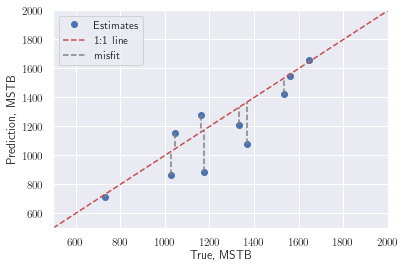Below the uncertainty models are shown along with the true values.

In :
create_realizations_plots(solution_df, truth_data)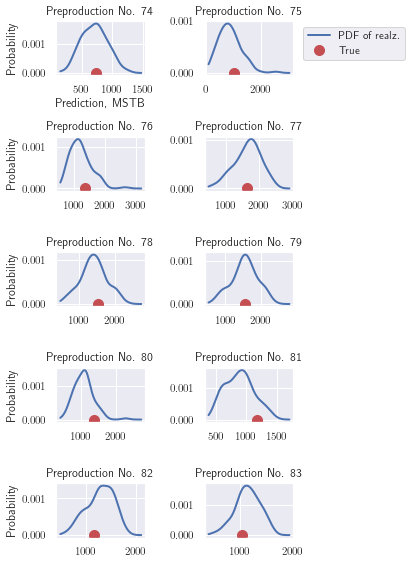And finally the goodness plot. The goodness measure we used in our scorer function above is related to the integral of the black dashed line with respect to the diagonal red line where the area below above the red line is weighted twice the area below.

In :
goodness = create_goodness_plot_and_return_goodness_score(solution_df,
truth_data);
print(f'Goodness: {goodness}')

Goodness: 0.856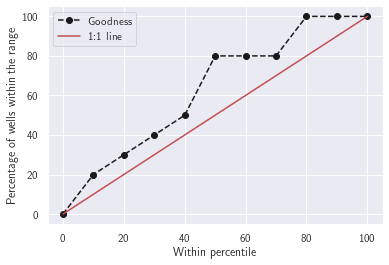Finally, my rank in the competition among the other teams (note, this is only for the objective part of the scoring, in the actual Hackathon, there we had additional columns for code quality and presentations, therefore the final rankings of the other teams in this table does not reflect the actual final outcome of the competition). Not bad for a days work!

In :
from IPython.display import Image
Image('results_table.png', width=500)

Out: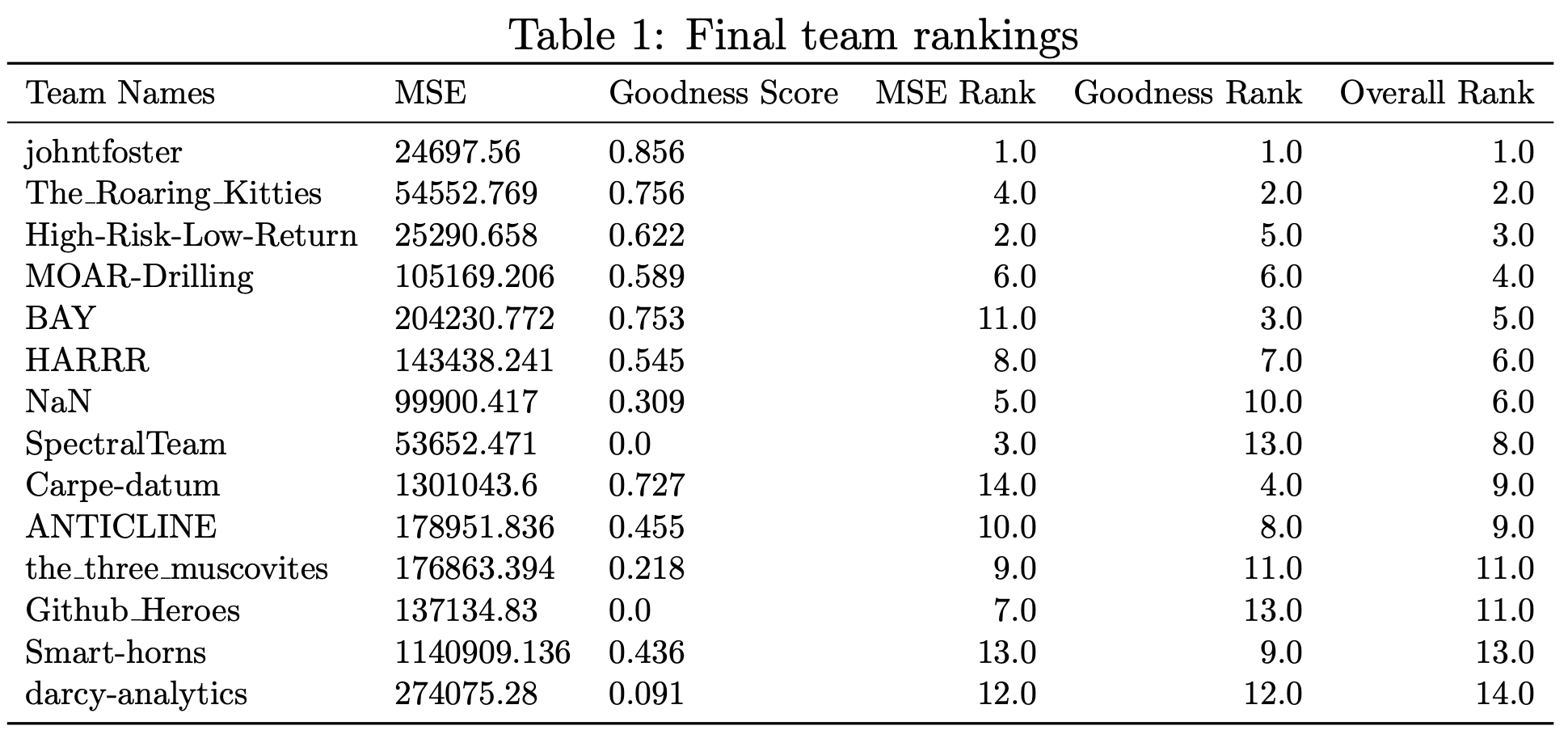The final message here is that domain expertise, which leads to good imputation and feature engineering strategies is far more useful than fancy machine learning models. Additionally, understanding exactly the quantities of interest you are looking for so that you evaluate (i.e. score) your models well. In the end, I "won" the competition with a linear regression model.

# JAX implementation of FEA and efficient inverse problem solving with neural networks

If you haven't heard by now JAX is getting a lot of attention online as a "NumPy on steroids". At it's core, it can be thought of as a drop-in replacement for NumPy where the array calculations can be accelerated on GPUs or TPUs when available. This alone makes it worth looking at, especially if you have a lot of NumPy code that you would like to potentially speed up with GPU acceleration. Currently, most of the NumPy API is implemented in one-to-one correspondence, as well of some of the most used functions in SciPy.

The accelerated NumPy is just the beginning of the utility of JAX. All of the JAX NumPy data structures can be used in combination with most pure Python code to create functions which can be automatically differentiated. This includes computing the gradient of scalar functions, as well as Jacobian matrices of vector functions. These operations can be composed to compute gradients-of-gradients, etc. More information of the automatic differentiation capabilities are documented here.

Additionally, there is a built-in just-in-time compiler for compiling functions to be executed on CPUs/GPUs/TPUs, and support for automatic vectorization, i.e. functions written for scalar arguments can be easily mapped across arrays. These can be used with the automatic differentiation functions previously mentioned.

Finally, there is a very thin neural network library associated with JAX called stax. Other, more fully-featured libraries like Haiku, Flax, or Trax are under development on top of JAX technologies.

In what follows, I'll highlight most of these features of JAX by implementing a finite element analysis (FEA) model and then using the finite element residual as part of the objective function when training a neural network in an inverse solution to a potentially unknown constitutive model.

### Problem definition¶

As a model problem, we'll start with the one-dimensional pressure diffusivity equation which governs single phase fluid flow in a porous media with fluid density $\rho$ and small compressibility $c$.

$$\rho c \frac{\partial p}{\partial t} = \frac{\partial}{\partial x}\left(\frac{\kappa}{\mu} \frac{\partial p}{\partial x}\right)$$

Assuming steady state, multiplying by a test function $\delta p$ on the left and integrating by parts over the domain $(0, L)$ we have

\begin{align} 0 =& \int_0^L \lambda(x) \frac{\partial \left(\delta p\right)}{\partial x} \frac{\partial p}{\partial x} \textrm{d}x - \left[ \lambda(x) \delta p \frac{\partial p}{\partial x} \right]_0^L \\ =& \int_0^L \lambda(x) \frac{\partial \left(\delta p\right)}{\partial x} \frac{\partial p}{\partial x} \textrm{d}x - \left[ q \right]_0^L \end{align}

where

$$\lambda(x) = \frac{\kappa}{\mu},$$

$\kappa$ is the porous medium's permeability and $\mu$ is the fluid viscosity. $\lambda$ is known as the mobility and is assumed to be spatially varying.

Using a Galerkin appoximation, i.e. $p = N_J p_J$ and $\delta p = N_I$ for $I,J = 1, 2, \ldots$ basis functions and splitting the domain into $n$ intervals, we now have

\begin{align} 0 =& \sum_{k=0}^n p_J \left(\int_{x_k}^{x_{k+1}} \lambda(x) \frac{\partial N_I}{\partial x} \frac{\partial N_J}{\partial x} \textrm{d}x - \left[ q \right]_{x_k}^{x_{k+1}} \right) \end{align}

where summation over the $J$ basis functions are implied for those that have support on the $I^{th}$ node. The right-hand side above is our residual, i.e. $\vec{R}$

\begin{align} R_I \equiv \sum_{k=0}^n p_J \left(\int_{x_k}^{x_{k+1}} \lambda(x) \frac{\partial N_I}{\partial x} \frac{\partial N_J}{\partial x} \textrm{d}x - \left[ q \right]_{x_k}^{x_{k+1}} \right) \end{align}

below, we'll integrate this residual vector using Gauss integration and solve for the unknown nodal pressures $p_J$. Without loss of generality we'll only consider Dirchelet boundary conditions, i.e. $q(x) = 0$.

While this model problem is linear, we'll implement the FEA model to use the residual form of the equations, and solve for the unknowns using a nonlinear Newton-Raphson solver where the Jacobian matrix at each iteration is computed via automatic-differentiation with JAX. All of the computations are written in a way that they could be accelerated on GPUs/TPUs and are just-in-time compiled.

### FEA implementation¶

Below are the imports we need, note that we explicitly enable 64-bit floating point numbers for JAX as 32-bit is the default.

In :
from functools import partial, partialmethod

import numpy as np
import matplotlib.pyplot as plt

from jax import jit, vmap, value_and_grad, random, flatten_util
import jax.numpy as jnp
import jax.scipy.optimize
import jax.ops
from jax.config import config
config.update("jax_enable_x64", True)

In :
class FEAProblem():

def __init__(self, nodes, mobility=lambda x: 1, left_bc=1.0, right_bc=-1.0):
"""FEAProblem class

Args:

nodes (array-like): nodal spatial locations of unknowns
mobility (callable): function defining the mobility function
in space
left_bc (real): Dirchelet boundary on the left of the domain
right_bc (real): Dirchelet boundary on the right of the domain
"""

self.nodes = jnp.array(nodes, dtype='float64')
self.connect = jnp.array([ jnp.arange(i,i+2)
for i in range(self.nodes.shape - 1)])
self.__lbc = left_bc
self.__rbc = right_bc
self.mobility = mobility

return

def setup_element_matrices(self):
"""Integrates the element stiffness matrices for linear basis functions
with 2 points Guass integration
"""

nodes = self.nodes

#We will use Gauss integration at the following points
t1 =  np.sqrt(1.0 / 3.0)
t2 = -np.sqrt(1.0 / 3.0)

# Because the points above are defined on the domain -1 < x < 1 and our
# elements are defined on arbitrary domains, we can use a change of
# variables to rescale the integration bounds
ξ1 = ((nodes[1:] - nodes[:-1]) * t1 + nodes[1:] + nodes[:-1]) / 2.0
ξ2 = ((nodes[1:] - nodes[:-1]) * t2 + nodes[1:] + nodes[:-1]) / 2.0

# Compute the function 𝜆, at the integration points
𝜆ξ1 = self.mobility(ξ1)
𝜆ξ2 = self.mobility(ξ2)

# Since the derivatives of the shape functions are not dependent on ξ,
# we can # create an array containing all of the element stiffness
# matrices at once
dNdξ = jnp.array([1 / (nodes[:-1] - nodes[1:]),
1 / (nodes[1:] - nodes[:-1])])
# Computes the matrix dN_i * dN_j for every element (each will be
# identical for equally spaced nodes)
ke_temp = jnp.einsum('i...,j...', dNdξ, dNdξ)

# Now we perform the Gauss integration computing the integrand for each
# element
temp = (nodes[1:] - nodes[:-1]) / 2 * (𝜆ξ1 + 𝜆ξ2)

# We have to add two axis so the broadcasting is performed correctly,
# the result here is the fully integrated element matrix array
self.ke = temp[:, None, None] * ke_temp

return

@partial(jit, static_argnums=(0,))
def residual(self, p):
"""Compute the FE residual vector

Args:
p (array-like): possible solution to residual equations

Returns:
(array-like): the residual vector
"""

nodes = self.nodes
connect = self.connect
ke = self.ke

# Initialize the residual
res = jnp.zeros_like(nodes)

# Compute the residual, i.e. sum all of the element
# matrices with the nodal values, p
res_temp = jnp.einsum('...ij, ...j', ke, p[connect]).flatten()

# This is the JAX equivalent of
# res[-1:1] = ...,  i.e. in place assignment
res = res.at[1:-1].set(res_temp[1:-1].reshape(-1, 2).sum(axis=1))
# Fix the residual values at the boundaries
res = res.at.set(p - self.__lbc)
res = res.at[-1].set(p[-1] - self.__rbc)

return res

@partial(jit, static_argnums=(0,))
def loss(self, p):
"""Compute the loss function, i.e. the
l2 norm of the residual vector.

Args:
p (array-like): possible solution to the residual equations

Returns:
(real): discrete 2-norm of residual vector
"""
return jnp.linalg.norm(self.residual(p))

@partial(jit, static_argnums=(0,))
def newton_step(self, p):
"""Compute one step of a Newton-Raphson iteration, uses
JAX to compute the exact Jacobian via automatic differentiation

Args:
p (array-like): possible solution to the residual equations

Returns:
p (array-like): updated solution to the residual equations after
a single Newton-Raphson step
"""

# The jacobian
K = jax.jacfwd(self.residual)(p)

# Compute the update direction
Δp, _ = jax.scipy.sparse.linalg.gmres(lambda x: -K @ x,
self.residual(p))

# Update the unknowns and return
p += Δp

return p

@partial(jit, static_argnums=(0,))
def solve(self, tolerance=1.0e-4):
""" Solve via Newton-Raphson iteration

Args:
tolerance (float): the tolerence at which the Newton-Raphson
iteration stops

Returns:
p (array-like): the converged solution to the residual equations
"""

# Integrate the shape functions over each element
self.setup_element_matrices()

# Initial guess is linear between the boundary conditions
p = jnp.linspace(self.__lbc, self.__rbc, num=self.nodes.shape)

# The Newton-Raphson loop, can be compiled and automatically
# differentiated
p = jax.lax.while_loop(lambda x: self.loss(x) > tolerance,
self.newton_step, p)

# Return the solution
return p


### Generate reference data¶

Below we'll solve the forward problem via FEA using the implementation above to verify things are working correctly as well as generate some reference data that we'll use in the inverse problem in the sequal. Here, the mobility function is

$$\lambda(x) = x^3 + 0.001$$
In :
nodes = jnp.linspace(0, 1, num=20)
problem1 = FEAProblem(nodes, mobility = lambda x: x ** 3 + 0.001,
left_bc=15, right_bc=5)
p1 = problem1.solve(tolerance=1.0e-4)

WARNING:absl:No GPU/TPU found, falling back to CPU. (Set TF_CPP_MIN_LOG_LEVEL=0 and rerun for more info.)

In :
fig, ax1 = plt.subplots()
ax2 = ax1.twinx()
ax1.plot(nodes, p1, 'k')
ax2.plot(nodes, nodes ** 3 + 0.001, 'r-.')
ax1.set_xlabel(r'$x$')
ax1.set_ylabel(r'$p(x)$', color='k');
ax2.set_ylabel(r'$\lambda(x)$', color='r');
ax2.tick_params(axis='y', colors='r')
ax2.spines['right'].set_color('r')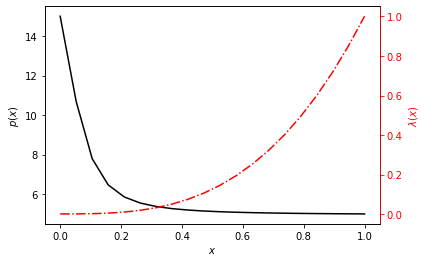### Inverse problem implementation¶

Here we will write our inverse problem solver. We will inherit from the FEAProblem class above so we can reuse some of the functions already defined.

Our objective function here will be the $l_2$-norm of the finite element residual when the "known data" is supplied as training data. Because the problem we are solving is a steady-state problem, we'll need to provide the endpoints of constitutive model to the objective function, otherwise there are infinite valid solutions to learning the constitutive model that only differ by a constant. If we extended this technique to time-dependent problems, I believe the need to provide the boundary constraints can be avoided.

We'll use a few functions from the jax.experimental.stax module, just to make the neural network construction easier. Our minimizer here will use the second-order "BFGS" method from jax.scipy.optimize.minimize.

Here we assume our data is supplied at the nodes of the FE model, but this restriction could be easily generalized by evaluating the residuals at any given spatial location via the FE shape functions.

In :
from jax.experimental import stax
from jax.experimental.stax import Dense, Tanh
from jax.nn.initializers import glorot_normal, normal

In :
class InverseProblem(FEAProblem):

def __init__(self, nodes, mobility = stax.serial(Dense(1)),
mobility_left_bc=0.001, mobility_right_bc=1.001):
"""Class to solve for (i.e. learn) the constitutive model of
the steady-state pressure diffusivity equation using a
neural network (nn).

Args:
nodes (array-like): the spatial locations corresponding to the
data points
mobility (stax.nn): a stax neural network definition
mobility_left_bc (real): the left boundary value of the
mobility function
mobility_right_bc (real): the right boundary value of the
mobility function
"""
super().__init__(nodes, mobility = mobility)

self.__mlbc = mobility_left_bc
self.__mrbc = mobility_right_bc

# unpack the functions to initialize and apply the network parameters
net_init, self.net_apply = self.mobility

# initialize the network parameters with random numbers
seed = random.PRNGKey(10)
_, net_params = net_init(seed, (-1, 1))

# flatten the nn parameters into an single array
self.initial_net_params_flat, self.unravel_pytree = flatten_util.ravel_pytree(net_params)

@partial(jit, static_argnums=(0,))
def residual(self, u, data):
"""Compute the FE residual vector

Args:
u (array-like): the unknown parameters of the nn
data (array-like): the user supplied data (i.e. pressures)
at the nodes

Returns:
(array-like): residual vector
"""

# Put the parameters back into the stax nn data structure
net_params = self.unravel_pytree(u)

# Set the mobility function to be the current nn
self.mobility = lambda x: self._predict(x, net_params)
mobility = self.mobility(self.nodes)

# Integrate the element shape functions with the current
# mobility function
self.setup_element_matrices()

# Return the FE residual vector with the supplied data
return super().residual(data)

@partial(jit, static_argnums=(0,))
def loss(self, u, data):
"""Compute the squared error of the FE residual vector
along with the mobility at the end points

Args:
u (array-like): the unknown parameters of the nn
data (array-like): the user supplied data (i.e. pressures) at
the nodes

Returns:
(real): squared error of the FE residual and mobility function
end points
"""

# Compute FE residual with supplied data
residual = self.residual(u, data)

# Put the unknowns back into the stax nn data structure
net_params = self.unravel_pytree(u)

# Use the nn parameters to compute the mobility at the nodes
mobility = self._predict(nodes, net_params)

# Compute squared error norm of mobility end points
mobility_squared_error = ((mobility - self.__mlbc) ** 2 +
(mobility[-1] - self.__mrbc) ** 2)

# Return FE + mobility squared error
return jnp.linalg.norm(residual) ** 2 + mobility_squared_error

def fit(self, data):
"""Fit the parameters of the neurual network representing the mobility
function

Args:
data (array-like): known pressure data at the nodal locations
"""

self.__lbc = data
self.__rbc = data[-1]

u0 = self.initial_net_params_flat

# minimize the loss function w.r.t. the uknown parameters of the nn
u = jit(lambda u, data: jax.scipy.optimize.minimize(self.loss, u,
args=(data,),
method='BFGS').x)(u0, data)

# assign the final nn params, such that we can use if for predicting
self.net_params = self.unravel_pytree(u)

return

def _predict(self, x, params):
"""Used internal to the class only - evaluates the nn function
at x with the given parameters
"""
return vmap(partial(self.net_apply, params))(x).flatten()

def predict(self, x):
"""Evaluates the nn function at x with the fit parameters

Args:
x (array-like): spatial locations to evalute the neural network
"""
return self._predict(x, self.net_params)


### Solve inverse problem using NN¶

Below we'll test out our inverse problem solver using the data generated earlier from the forward finite element solution. First we define our neural network architecture. This is a fairly simple function, so we don't need a large and/or complex neural network. Here we have an input layer with only 4 nodes and a $\tanh$ activation function feeding to a single node output. More complicated architectures also work, yielding the same result at more computational cost.

We also need to define the layer Dense64 which is the same as stax.Dense, but initialized to use 64-bit floats to be consistant with our data structures in the FEA residual calculation.

In :
Dense64 = lambda x: Dense(x, W_init=glorot_normal(dtype='float64'),
b_init=normal(dtype='float64'))

nn = stax.serial(
Dense64(4), Tanh,
Dense64(1)
)


Now we instantiate the model and solve the inverse problem, i.e. train the network. We do have to supply the endpoints of the constitutive model. Given the problem is parabolic, there are infinite solutions to the inverse problem (they all have the same shape, but differ by a constant scale factor). We could remove this restriction by considering a time-dependent problem and supplying the time depended training data which we'll leave for future work.

In :
iproblem1 = InverseProblem(nodes, mobility = nn,
mobility_left_bc = 0.001, mobility_right_bc = 1.001)
iproblem1.fit(p1)


Plotting the neural network function over the range of the domain and comparing with the reference, we can see that the inverse solver has "learned" the mobility function well.

In :
x = nodes
fig, ax = plt.subplots()
ax.plot(x, x ** 3 + 0.001, 'k-')
ax.plot(x, iproblem1.predict(x), 'r-.')
ax.set_xlabel(r'$x$')
ax.set_ylabel(r'$\lambda(x)$');
ax.legend([r'$\lambda(x) = x^3 + 0.001$', r'$\lambda(x) = \mathcal{NN}(x)$']);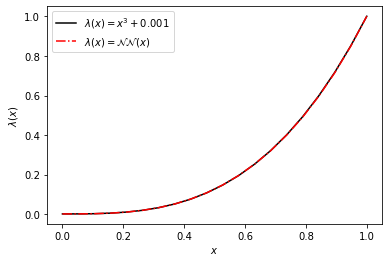### Use the trained NN in the forward problem (verification)¶

Just to verify, we'll use our neural network as the mobility function in our forward finite element solver to demonstrate the resulting pressures are also accurate.

In :
vproblem1 = FEAProblem(nodes, mobility = iproblem1.predict,
left_bc=15, right_bc=5)
v1 = vproblem1.solve()
fig, ax = plt.subplots()
ax.set_xlabel(r'$x$')
ax.set_ylabel(r'$p(x)$');
ax.plot(nodes, p1, 'k-');
ax.plot(nodes, v1, 'r-.');
ax.legend([r'$\lambda(x) = x^3 + 0.001$', r'$\lambda(x) = \mathcal{NN}(x)$']);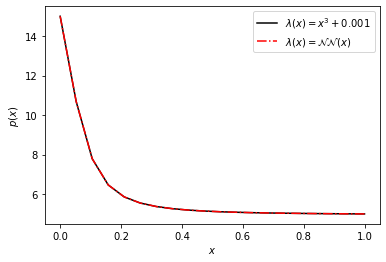### Validate for different BCs¶

A major advantage of this approach over say, physics-informed neural networks, is that we have only "learned" the constitutive model, i.e. the mobility function, not the solution of the partial differential equation with the supplied boundary conditions. Instead, we rely on our finite element implementation to compute the solution. Which means we can now use our "learned" constitutive model to solve problems with different boundary conditions accurately.

In :
problem2 = FEAProblem(nodes, mobility = lambda x: x ** 3 + 0.001,
left_bc=5, right_bc=20)
p2 = problem2.solve(tolerance=1.0e-4)
problem3 = FEAProblem(nodes, mobility = iproblem1.predict,
left_bc=5, right_bc=20)
p3 = problem3.solve(tolerance=1.0e-4)
fig, ax = plt.subplots()
ax.plot(nodes, p2, 'k-');
ax.plot(nodes, p3, 'r-.');
ax.legend([r'$\lambda(x) = x^3 + 0.001$', r'$\lambda(x) = \mathcal{NN}(x)$']);